1. The first graph plots data from a soap density measurement laboratory. The slope of the line is the density of the soap.
1. __________ Calculate the slope of the line.
2. ______________ Determine the y-intercept of the line.
3. y = _________ x + __________ Write the y = mx + b slope-intercept equation for the line.
4. [float] or [sink] The slope of the line is the density of soap. If the density of the soap is less than one gram/centimeter³ then the soap would float. If the density of the soap is greater than one gram/centimeter³ then the soap would sink. Based on the above, will this soap float or sink?
2. A student gathered the data seen in the table below. Plot the data on the graph.
1. __________ Calculate the slope of the line.
2. __________ Calculate the intercept of the line.
3. __________ cm. How far will the RipStik travel in 23 seconds?
4. __________ seconds. How long in seconds for the RipStik to travel 7500 centimeters?
3. __________ What is the slope of the line y = 2.0 + 0.15x?
4. __________ What is the y-intercept of the line y = 2.0 + 0.15x?
5. __________ __________ For the Desmos graph and regression, determine the acceleration of gravity g.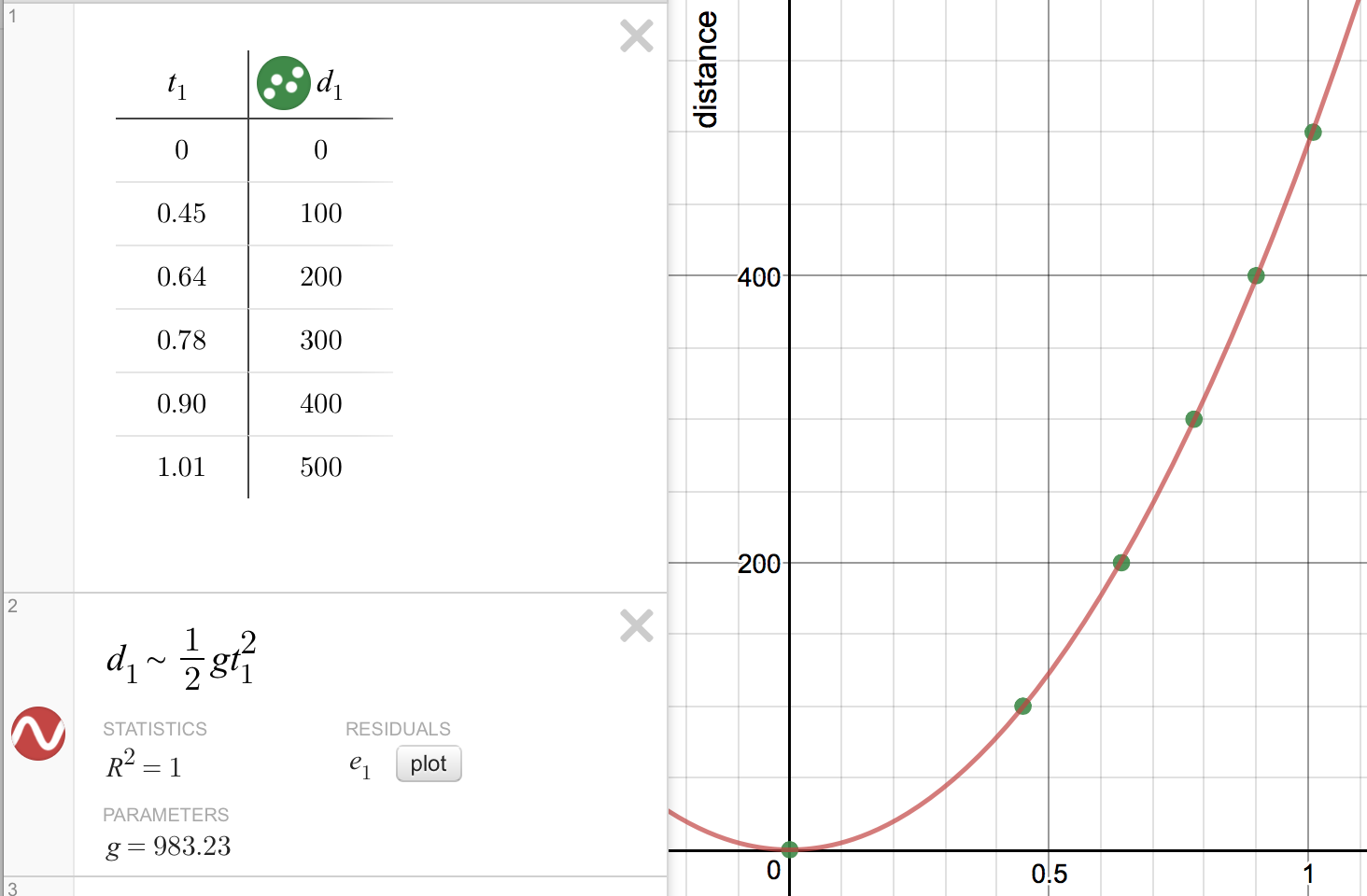6. The Desmos graph shows force of friction f data for weight w1, surface area s2, and grit g3.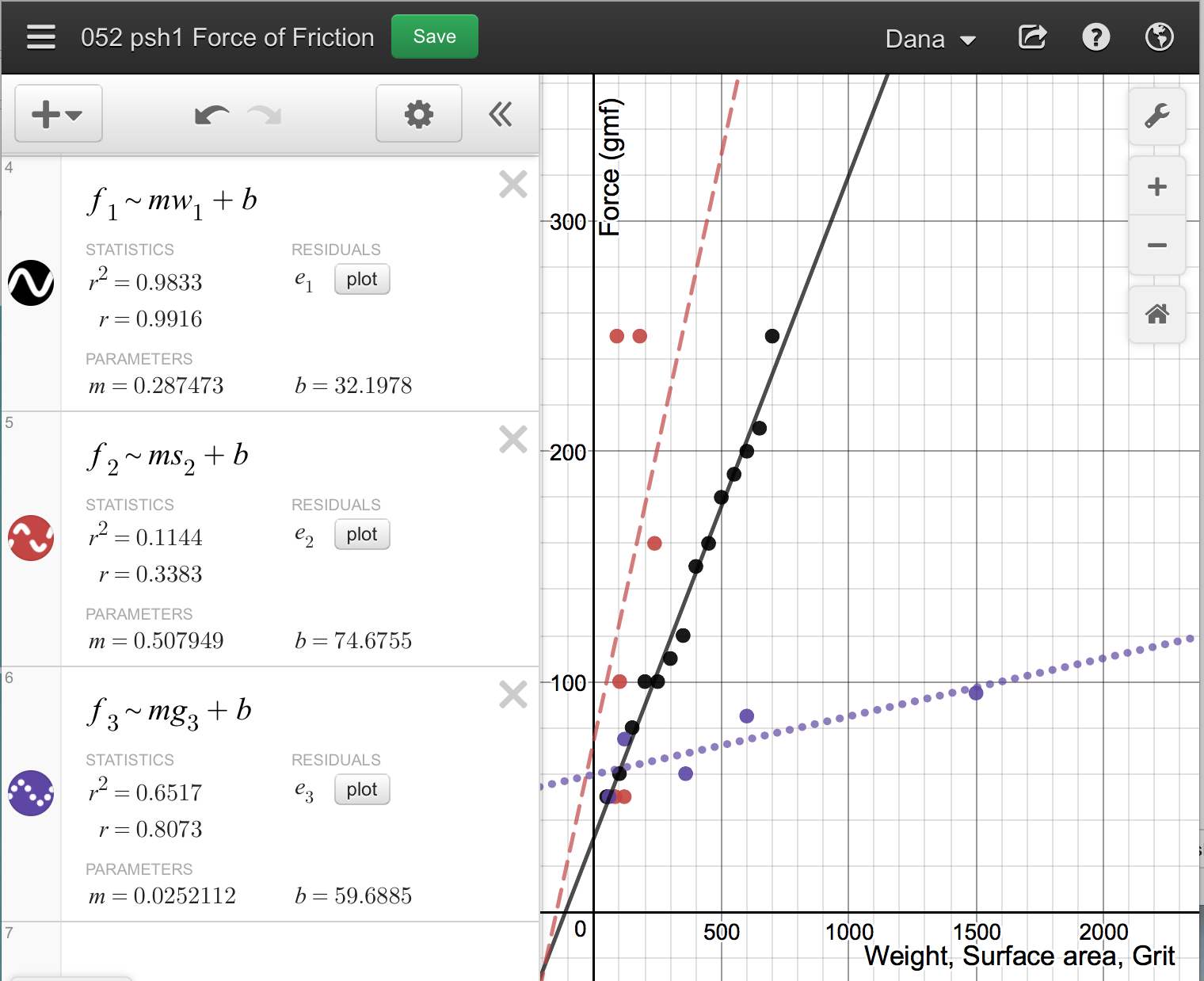1. _______________ Based on the data above, which variable has the most predictable effect on the force of friction?
2. Why?
3. _______________ Based on the data above, which variable has the least predictable effect on the force of friction?
4. Why?
7. A student rolled marbles into a line of five marbles.
1. ______________ What will be the slope of the graph for marbles in versus marbles out?
2. _____________________ ____ ________________ What law of motion are the marbles demonstrating?
8. A wave was inscribed on poster paper by a RipStik and the plotted in Desmos.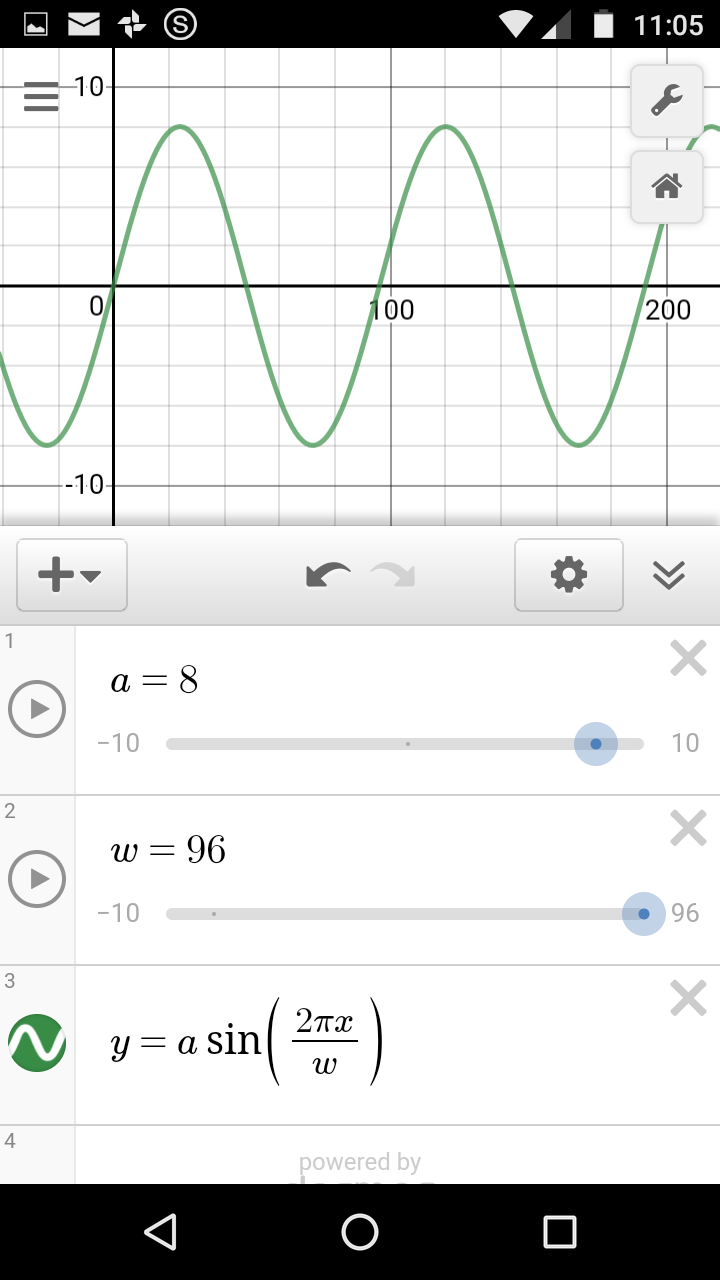1. λ = _________ _________ What is the wavelength λ of ONE wave?
2. a = _________ _________ What is the amplitude a?
3. τ = _________ _________ The RipStik took 1.45 seconds to swizzle (wiggle) 2.5 times. What is the period τ of ONE wave?
4. f = _________ _________ What is the frequency f of the RipStik wave?
5. ѵwave = _________ _________ What is the wave velocity ѵwave?
9. Explain what happens to sea surface temperatures in the western Pacific during an El Niño.
10. Explain what happens to precipitation in the western Pacific during an El Niño.
11. With respect to storms in the western Pacific, what is the "El Niño, box"?
12. List the three primary colors of light:
13. Kestrels (a type of hawk related to falcons) use light to determine if a field has voles (related to mice) in a field. What is the name of the type of light the kestrel is using and how are they using that light?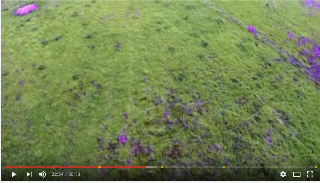14.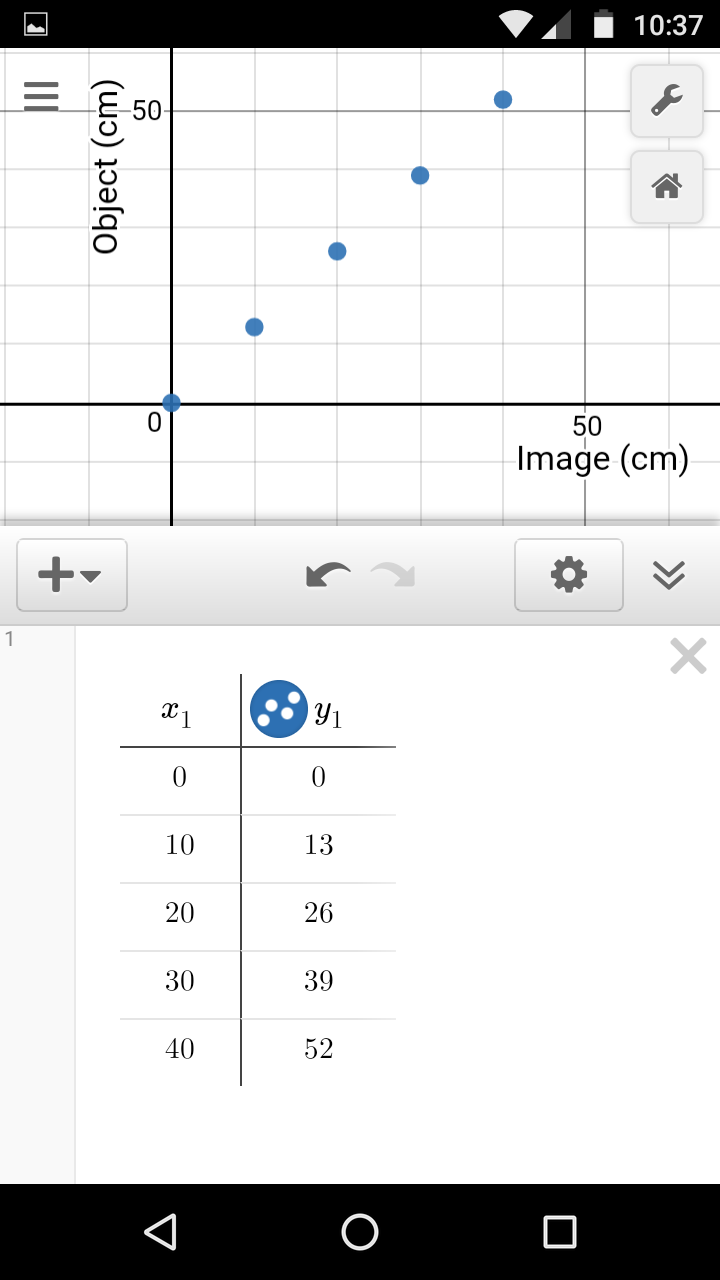__________ Based on the image at the right (maybe on next page) where x1 is the image depth and y1 is the object depth for an object underwater, what is the index of refraction for water?
1. ______________ __________ Use the index of refraction calculated above to predict the object depth for a penny that appears to be 30 cm underwater.
2. ______________ __________ Use the index of refraction calculated above to predict the image depth for a coral head that is 200 cm underwater.
3. ______________ Water has a published index of refraction of 1.33. What is the error for the slope?
4. ______________ Water has a published index of refraction of 1.33. What is the percentage error for the slope?
15. A Whirlpool dryer uses 26 amps of current at 240 volts.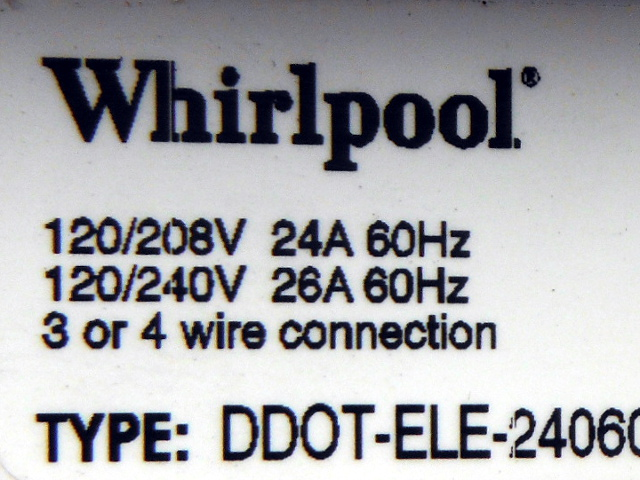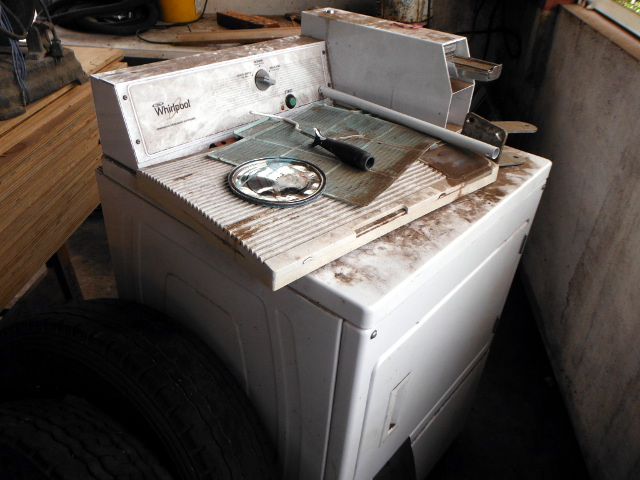1. __________ _____ Given that V = iR, calculate the resistance R for the dryer.
2. __________ _____ Given that P = iV, calculate the power P for the dryer.
3. __________ Power is now forty cents per kiloWatt hour, or \$0.40 for 1000 Watts for one hour. How much does it cost to run the dryer for one hour?
16. [Note that in an atom the number of neutrons is equal to or greater than the number of protons. Thus the more numerous nucleons are the neutrons.]
1. _______________ Looking only at the atomic diagram on this paper, determine the atomic number of the atom depicted.
2. _______________ Looking at the diagram, what is the atomic mass for the atom?
3. _______________ Looking at the diagram and the chart on the wall, what is the one or two letter chemical abbreviation for this element?
4. _______________ What is the full name for this element?
17.Cloud type: ______________________
18.Cloud type: ______________________
19.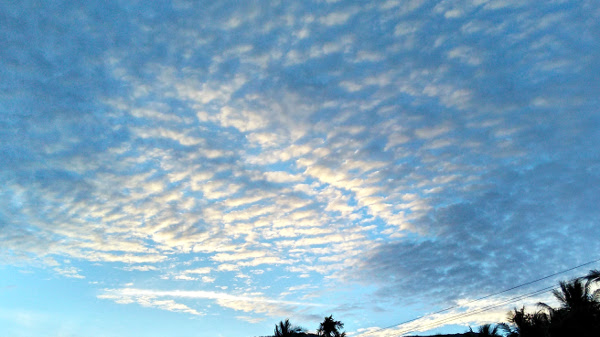Cloud type: ______________________
20. Data was collected on the time for marbles to roll down a ramp. The data recorded is the marble weight w1 in gram-force versus the time t1 in second to roll down the ramp.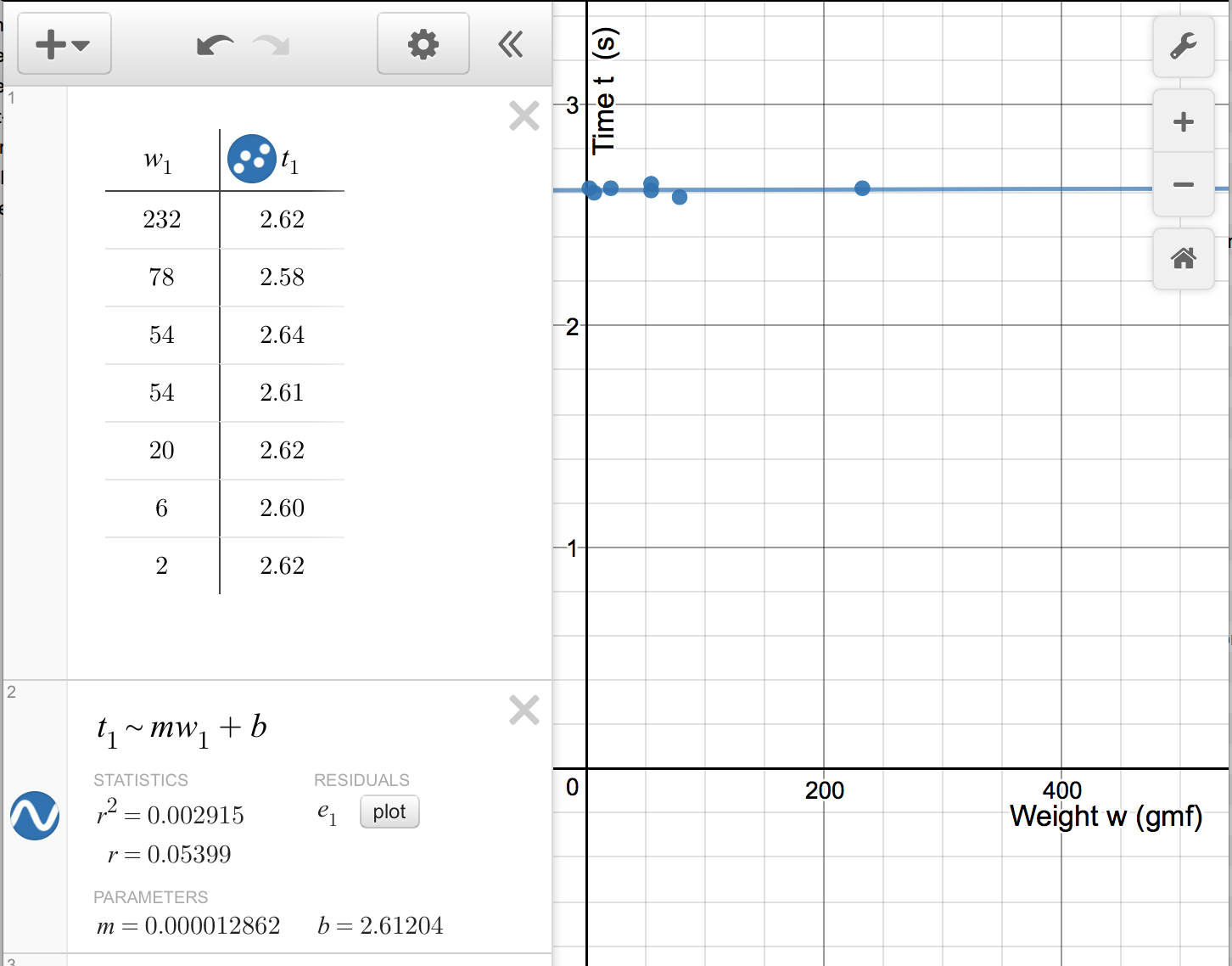1. ____________ __________ What is the slope of the relationship?
2. Based on the experiment, does the time for a marble to roll down a ramp depend on the weight of the marble? Why?

$\text{slope m}=\frac{\left({y}_{2}-{y}_{1}\right)}{\left({x}_{2}-{x}_{1}\right)}$
raw error = experimental value − expected value
$\text{percent error}=\frac{\left(\text{experimental value}-\text{expected value}\right)}{\left(\text{expected value}\right)}$
period τ = 1 ÷ (frequency f)
frequency f = 1 ÷ (period τ )
velocity ѵ = wavelength λ × frequency f